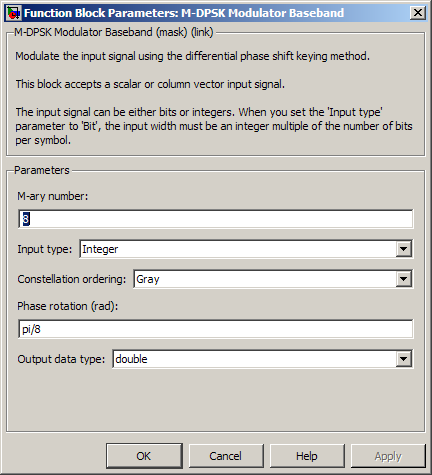# M-DPSK Modulator Baseband

Modulate using M-ary differential phase shift keying method

## Library

PM, in Digital Baseband sublibrary of Modulation

•## Description

The M-DPSK Modulator Baseband block modulates using the M-ary differential phase shift keying method. The output is a baseband representation of the modulated signal. The M-ary number parameter, M, is the number of possible output symbols that can immediately follow a given output symbol.

The input must be a discrete-time signal. For integer inputs, the block can accept the data types `int8`, `uint8`, `int16`, `uint16`, `int32`, `uint32`, `single`, and `double`. For bit inputs, the block can accept `int8`, `uint8`, `int16`, `uint16`, `int32`, `uint32`, `boolean`, `single`, and `double`.

The input can be either bits or integers, which are binary-mapped or Gray-mapped into symbols.

This block accepts column vector input signals. For a bit input, the input width must be an integer multiple of the number of bits per symbol.

### Integer-Valued Signals and Binary-Valued Signals

If you set the Input type parameter to `Integer`, then valid input values are integers between 0 and M-1. In this case, the input can be either a scalar or a frame-based column vector. If the first input is k1, then the modulated symbol is

`$\mathrm{exp}\left(j\theta +j2\pi \frac{{k}_{1}}{m}\right)$`

where θ represents the Phase rotation parameter. If a successive input is k, then the modulated symbol is

When you set the Input type parameter to `Bit`, the block accepts binary-valued inputs that represent integers. The block collects binary-valued signals into groups of K = log2(M) bits

where

K represents the number of bits per symbol.

The input vector length must be an integer multiple of K. In this configuration, the block accepts a group of K bits and maps that group onto a symbol at the block output. The block outputs one modulated symbol for each group of K bits.

The input can be a column vector with a length that is an integer multiple of K.

In binary input mode, the Constellation ordering parameter indicates how the block maps a group of K input bits to a corresponding phase difference. The `Binary` option uses a natural binary-to-integer mapping, while the `Gray` option uses a Gray-coded assignment of phase differences. For example, the following table indicates the assignment of phase difference to three-bit inputs, for both the `Binary` and `Gray` options. θ is the Phase rotation parameter. The phase difference is between the previous symbol and the current symbol.

Current InputBinary-Coded Phase DifferenceGray-Coded Phase Difference
[0 0 0]
[0 0 1] jθ + jπ/4 jθ + jπ/4
[0 1 0] jθ + jπ2/4 jθ + jπ3/4
[0 1 1] jθ + jπ3/4 jθ + jπ2/4
[1 0 0] jθ + jπ4/4 jθ + jπ7/4
[1 0 1] jθ + jπ5/4 jθ + jπ6/4
[1 1 0] jθ + jπ6/4 jθ + jπ4/4
[1 1 1] jθ + jπ7/4 jθ + jπ5/4

For more details about the `Binary` and `Gray` options, see the reference page for the M-PSK Modulator Baseband block. The signal constellation for that block corresponds to the arrangement of phase differences for this block.

## Dialog BoxM-ary number

The number of possible output symbols that can immediately follow a given output symbol.

Input type

Indicates whether the input consists of integers or groups of bits. If this parameter is set to `Bit`, then the M-ary number parameter must be 2K for some positive integer K.

Constellation ordering

Determines how the block maps each group of input bits to a corresponding integer.

The phase difference between the previous and current modulated symbols when the input is zero.

Output data type

The output data type can be either `single` or `double`. By default, the block sets this to `double`.

## Supported Data Types

PortSupported Data Types

Input

• Double-precision floating point

• Single-precision floating point

• Boolean (binary input mode only)

• 8-, 16-, and 32-bit signed integers

• 8-, 16-, and 32-bit unsigned integers

Output

• Double-precision floating point

• Single-precision floating point

## Pair Block

M-DPSK Demodulator Baseband

## References

 Pawula, R. F., "On M-ary DPSK Transmission Over Terrestrial and Satellite Channels," IEEE Transactions on Communications, Vol. COM-32, July 1984, 752-761.

## Version History

Introduced before R2006a